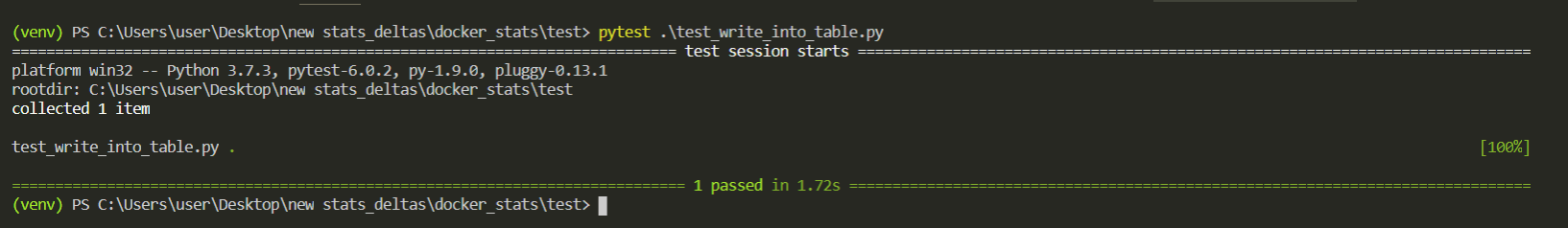GFG App
Open AppBrowser
Continue

# How to Mock AWS DynamoDB Services for Unit Testing?

In this article, we will be understanding how to mock out DynamoDB resources with the help of short examples. But before this, first, we have to make out why we use mocking for testing purposes? Mocking is something where we try to make a copy or replica of some resources that are required for testing purposes. Sounds confusing right? In simple terms we make a copy of something so that we can test each and every aspect of anything without having the fear of being lost or updated something important.

Let’s suppose we are having a function that writes the given data into the given DynamoDB table as shown below. This is the simplest method that we can take to illustrate the working of mocking AWS DynamoDB. In the given function, it uses DynamoDB resources to store the data. So, here we will be mocking the AWS DynamoDB with the help of the Moto Python module. Moto is very easy & convenient to implement.

## Python3

 `# store.py ` ` `  `def` `write(data, table_name): ` `   ``dynamodb ``=` `boto3.resource(``'dynamodb'``) ` `   ``table ``=` `dynamodb.Table(table_name) ` `   ``with table.batch_writer() as batch: ` `       ``batch.put_item(Item``=``data)`

For mocking this function we will use a few steps as follows –

• At first, build the skeleton by importing the necessary modules & decorating our test method with @mock_dynamodb2. Create a new file called test_write_into_table.py and add the following line:

## Python3

 `import` `boto3 ` `from` `moto ``import` `mock_dynamodb2 ` `import` `store ` `  `  `@mock_dynamodb2` `def` `test_write_into_table(): ` `   ``"Test the write_into_table with a valid input data"`

• Now, within the test_write_into_table() method, create a DynamoDB resource like following –
```dynamodb = boto3.resource('dynamodb')
```
• Let’s create a DynamoDB table using the DynamoDB resource like below-given code. There we created a table named ‘test’ with primary key ‘date’ of type string(‘S’).

## Python3

 `table_name ``=` `'test'` `table ``=` `dynamodb.create_table(TableName``=``table_name, ` `       ``KeySchema``=``[{``'AttributeName'``: ``'date'``,``'KeyType'``: ``'HASH'``}], ` `       ``AttributeDefinitions``=``[{``'AttributeName'``: ``'date'``,``'AttributeType'``: ``'S'``}])`

• Here, we will create input data to store.write(), which will also act as the expected data after reading.
```data = {'date' : '06-Nov-2020','organization' : 'GeeksforGeeks','articles' : 123456}
```
• For better understanding, we took single data but one can have more as per the requirement. After writing the above data into the table, the table will look as follows –
• At this point, when store.write() is executed, it should store the given input data to the corresponding given table.
```store.write(data,table_name)
```
• Let’s read the data by passing ‘date’ as the primary key. Note that we are getting a dictionary with a couple of keys, so for getting our data we have to fetch the value of the ‘Item’ key.
```response = table.get_item(Key={'date':data['date']})
actual_output = response['Item']
```
• Let’s assert that the body of the data is the same as we stored.
```assert actual_output == data
```

Complete Source Code:

## Python3

 `""" ` `Example of using moto to mock out DynamoDB table ` `"""` ` `  `import` `boto3 ` `from` `moto ``import` `mock_dynamodb2 ` `import` `store ` ` `  ` `  `@mock_dynamodb2` `def` `test_write_into_table(): ` `    ``"Test the write_into_table with a valid input data"` `    ``dynamodb ``=` `boto3.resource(``'dynamodb'``) ` `    ``table_name ``=` `'test'` `    ``table ``=` `dynamodb.create_table(TableName ``=` `table_name, ` `                                  ``KeySchema ``=` `[ ` `                                  ``{``'AttributeName'``: ``'date'``, ``'KeyType'``: ``'HASH'``}], ` `                                  ``AttributeDefinitions ``=` `[ ` `                                  ``{``'AttributeName'``: ``'date'``, ``'AttributeType'``: ``'S'``}]) ` `    ``data ``=` `{``'date'``: ``'06-Nov-2020'``, ` `            ``'organization'``: ``'GeeksforGeeks'``, ``'articles'``: ``123456``} ` `    ``store.write(data, table_name) ` `    ``response ``=` `table.get_item(Key``=``{``'date'``: data[``'date'``]}) ` `    ``actual_output ``=` `response[``'Item'``] ` `    ``assert` `actual_output ``=``=` `data `

Output :My Personal Notes arrow_drop_up